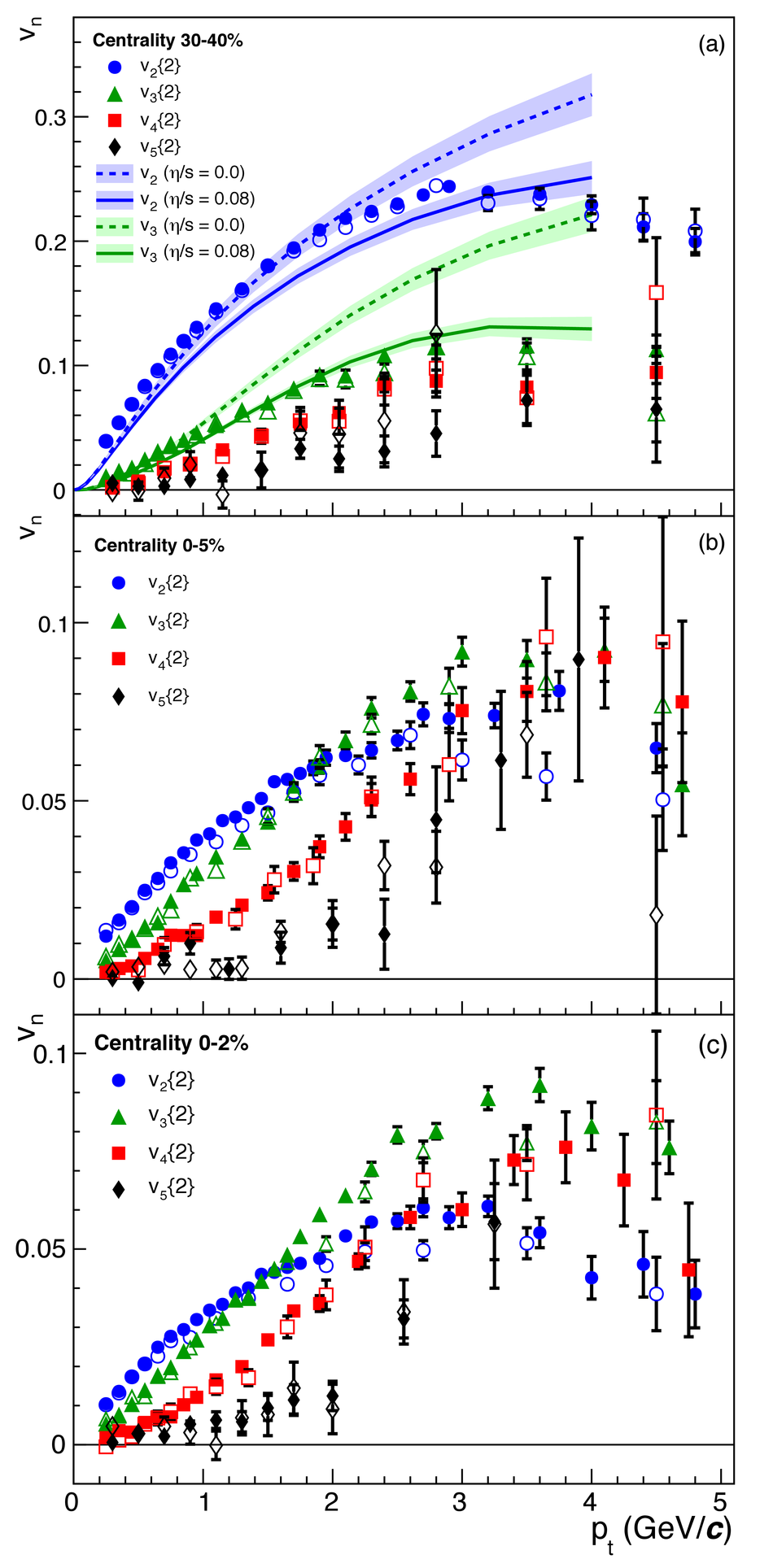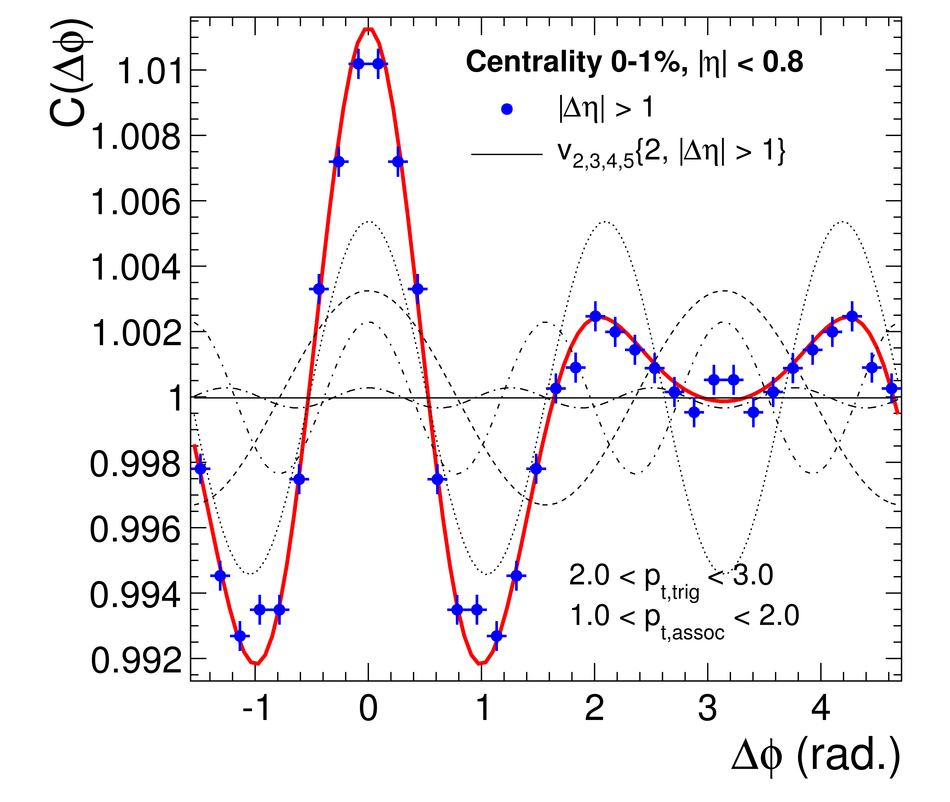Higher harmonic anisotropic flow measurements of charged particles in Pb-Pb collisions at $\sqrt{s_{\rm NN}} = 2.76~$ TeV

We report on the first measurement of the triangular v3, quadrangular v4, and pentagonal v5 charged particle flow in Pb-Pb collisions at $\sqrt{s_{\rm NN}} = 2.76~$ TeV measured with the ALICE detector at the CERN Large Hadron Collider. We show that the triangular flow can be described in terms of the initial spatial anisotropy and its fluctuations, which provides strong constraints on its origin. In the most central events, where the elliptic flow v2 and v3 have similar magnitude, a double peaked structure in the two-particle azimuthal correlations is observed, which is often interpreted as a Mach cone response to fast partons. We show that this structure can be naturally explained from the measured anisotropic flow Fourier coefficients.

Figures

Figure 1

 a) $v_2$, $v_3$ and $v_4$ integrated over the $\pt$ range $0.2 < \pt < 5.0$ GeV/$c$ as a function of event centrality, with the more central (peripheral) collisions shown on the left- (right-)hand side, respectively Full and open squares show $v_3${2} and $v_3${4}, respectively In addition we show $v^2_{3/\Psi_2}$ and $v_{3/\Psi_{\rm RP}}$, which represent the triangular flow measured relative to the second order event plane and the reaction plane, respectively (for the definitions, see text) b) $v_2${2, $|\Delta\eta| > 1$} and $v_3${2, $|\Delta\eta| > 1$} divided by the corresponding eccentricity versus centrality percentile for Glauber  and MC-KLN CGC  initial conditions.Figure 2

 $v_2$ and $v_3$ as a function of centrality for the 5\% most central collisions compared to calculations of the spatial eccentricities, $\varepsilon_{n}^W${2} and $\varepsilon_{n}^{\rm CGC}${2} The eccentricities have been scaled to match the 2-3% data using $k_1$ and $k_2$Figure 3

 $v_2$, $v_3$, $v_4$, $v_5$ as a function of transverse momentum and for three event centralities. The full, open symbols are for $\Delta\eta > 0.2$ and $\Delta\eta > 1.0$, respectively a) 30% - 40% compared to hydrodynamic model calculations b) 0 - 5% centrality percentile c) 0 - 2% centrality percentileFigure 4

 The two-particle azimuthal correlation, measured in $0 < \Delta\phi < \pi$ and shown symmetrized over $2\pi$, between a trigger particle with $2 < \pt < 3$ GeV/$c$ and an associated particle with $1 < \pt < 2$ GeV/$c$ for the 0 - 1% centrality class. The solid red line shows the sum of the measured anisotropic flow Fourier coefficients $v_2$, $v_3$, $v_4$ and $v_5$ (dashed lines).